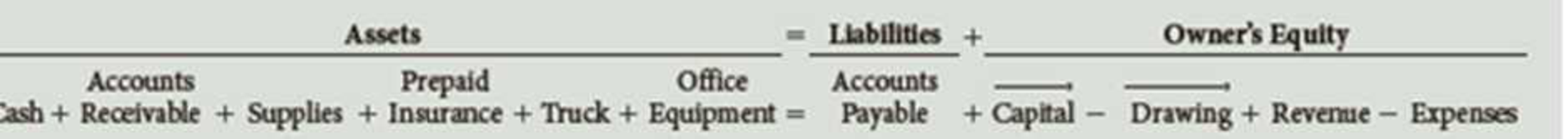Chapter 1, Problem 5PB

Chapter
Section
Textbook Problem

In October, A. Nguyen established an apartment rental service. The account headings are presented below. Transactions completed during the month of October follow.a. Nguyen deposited $25,000 in a bank account in the name of the business. b. Paid the rent for the month,$1,200, Ck. No. 2015. c. Bought supplies on account, $225. d. Bought a truck for$ 18,000, paying $1,000 in cash and placing the remainder on account e. Bought Insurance for the truck for the yean$1,400, Ck. No. 2016. f. Sold services on account $5,000. g. Bought office equipment on account from Henry Office Supply,$2,300. h. Sold services for cash for the first half of the month, $6,050. i. Received and paid the bill for utilities,$ 150, Ck. No. 2017. j. Received a bill for gas and oil for the truck. $80. k. Paid wages to the employees,$1,400, Ck Nos. 2018–2020. l. Sold services for cash for the remainder of the month, $4,200. m. Nguyen withdrew cash for personal use,$2,000, Ck. No. 2021. Required 1. Record the transactions and the balance after each transaction. 2. Total the left side of the accounting equation (left side of the equal sign), then total the right side of the accounting equation (right side of the equal sign). If the two totals are not equal, check the addition and subtraction. If you still cannot find the error, reanalyze each transaction.

1.

To determine

Record the figures in the given transactions for the respective accounts, and balance the figures after recording every transaction.

Explanation

Transaction: The economic events which bring about any changes in the financial items of a business and can be measured in the monetary units is referred to as a transaction.

Accounting equation: Accounting equation is a concept expressed in the form of equation, which creates a relation between resources or assets of a company and claims of resources to creditors and owners. Fundamental accounting equation is expressed as shown below:

Assets = Liabilities + Owners' EquityAssets = Liabilities+{(Owners' Capital

2.

To determine

Sum the balances of accounts to verify whether the balances on left side and on the right side in the accounting equation are equal.

Still sussing out bartleby?

Check out a sample textbook solution.

See a sample solution

The Solution to Your Study Problems

Bartleby provides explanations to thousands of textbook problems written by our experts, many with advanced degrees!

Get Started

Find more solutions based on key concepts